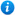# Solving Problems Involving Adding and Subtracting Fractions and Mixed Numbers - Year 6

Solving Problems Involving Adding and Subtracting Fractions and Mixed Numbers - Year 6

Year 6 maths programme of study - Number - fractions (including decimals and percentages)

Pupils are taught to:

• add and subtract fractions with different denominators and mixed numbers, using the concept of equivalent fractions
In this resource, pupils practise solving problems that involve adding and subtracting fractions and mixed numbers as per the curriculum objective of the year 6 maths programme of study listed above. This lesson supports the White Rose small steps guidance for year 6 - Autumn - Block 3 - Fractions. Content includes:

• Teaching PowerPoint presentation with class activities
• 3 differentiated worksheets with answers

'Solving Problems Involving Adding and Subtracting Fractions and Mixed Numbers - Year 6' is completely editable giving teachers the freedom to adapt the resource to suit their individual teaching needs.

Click on the images from the PowerPoint presentation to view the resource in more detail.

Our Price : £1.99 / 2 Credits